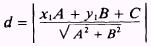# Find the equation of the line perpendicular to 2x-3y+5=0 which is at a distance of 5 units from the origin.PLEASE REPLY SOON.Thanks

12 years ago

Dear student,

slope of this line is  2/3

hence slope of perp line is -3/2

now find its equation....

12 years ago

Dear student

slope of the given line is = 2/3

slope of line perpendicular to this = -3/2

so the required equation of line is = y = -3x/2 + c

perpendicular distance from origin ==> d = 5 = | c/(13^1/2)|

=> c = + or - 5*(13^1/2)

i hope it explains